Classical Mechanics

# Free Body Diagrams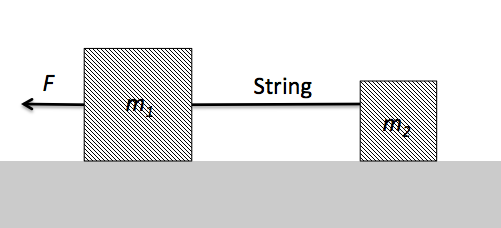The above diagram shows two blocks of respective masses $m_1 = 7 \text{ kg}$ and $m_2 = 2 \text{ kg}$ which are connected by a massless string and placed on a horizontal frictionless surface. If a force of $F = 27 \text{ N}$ is applied to $m_1,$ what is the force exerted by the string on $m_2?$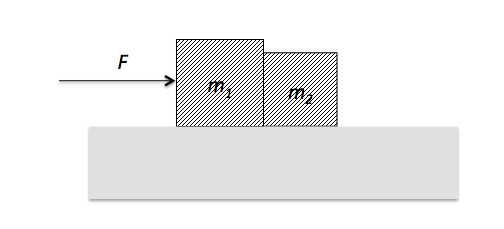Two blocks of respective masses $m_1 = 6 \text{ kg}$ and $m_2 = 3 \text{ kg}$ are placed in contact on a horizontal frictionless surface. If a force of $F = 27 \text{ N}$ is applied to mass $m_1,$ what is the contact force on $m_2?$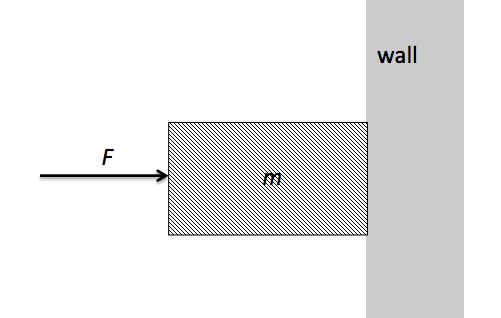A block of mass $m = 15 \text{ kg}$ is held stationary against a wall by applying a horizontal force $F$ on it, as shown in the above figure. If the coefficient of static friction between the wall and the block is $\mu_s = 0.3$ and the coefficient of kinetic friction is $\mu_k = 0.2,$ what is the minimum value of $F$ required to hold the block against the wall?

The gravitational acceleration is $g = 10 \text{ m/s}^2.$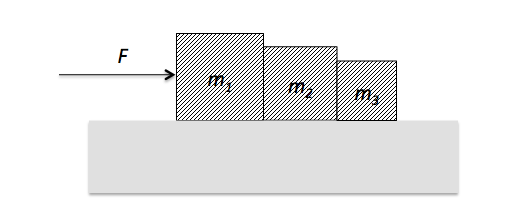Three blocks of respective masses $m_1 = 9 \text{ kg}, m_2 = 5 \text{ kg} \text{ and } m_3 = 2 \text{ kg}$ are placed in contact on a horizontal frictionless surface. If a force of $F = 16 \text{ N}$ is applied to $m_1,$ what is the contact force on $m_2$ (from $m_1$)?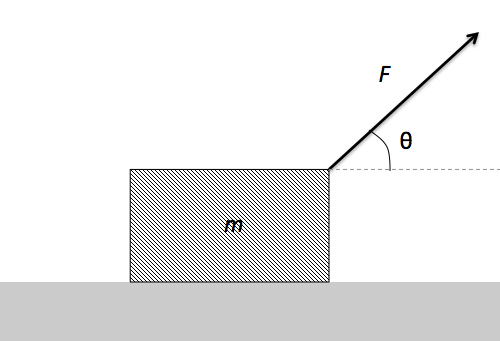A block of mass $m = 5 \text{ kg}$ is pulled by a force of $F = 30\sqrt{2} \text{ N}$ at an angle of $\theta = 45^\circ$ with the horizontal surface, as shown in the above figure. What is the acceleration of the block if the coefficient of kinetic friction between the surface and the block is $\mu = 0.2,$ assuming $g = 10 \text{ m/s}^2?$

×